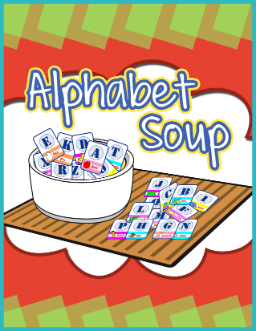# Alphabet Soup – Probability ActivityBegin by creating a set of cards with one letter of the alphabet on each card. Have the letters identified as vowels and consonants.

Put the cards inside a glass fishbowl or jar. Then ask students the following questions.

What is the probability of drawing a card that is a vowel? (The possible
outcomes are “a, e, i, o, u” so the probability is 5/26.

2)What is the probability of drawing a card that is a consonant? (the possible
outcomes are “b, c, d, f, g, h, j, k, l, m, n, p, q, r, s, t, v, w, x, y, z” so the
probability is 21/26.

3)What is the probability that either a consonant or a vowel will be chosen? (This
may stump students at first but they should see that the probability is 26/26 or
100%)

4)What is the probability that the letter drawn at random will be “r”? (the
probability is 1/26 that the letter will be “r”)

5)Which probability is higher? The probability that you’ll pick a vowel or a
consonant? (The probability that you’ll pick a consonant is more than four
times as high as the probability that you’ll pick a vowel.) 21/26 is over four
times as much as 5/26.

Which probability is higher? The probability that you select the “a”
card versus the probability that you select the “z” card?
(Both are equally probable, each has a probability of being selected
1 out of 26 or 1/26.)

What is the probability that the number 5 will be chosen?
(This may stump students at first until they realize that the answer is
0/26 or 0% probability.)